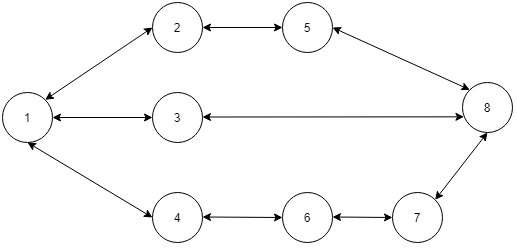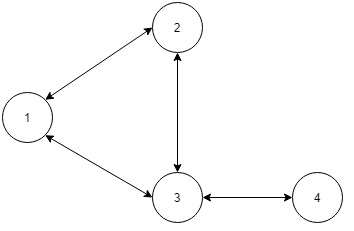Graphs Notes
Is it a Tree?
M-Coloring Problem
Count nodes within K-distance
Shortest path in an unweighted graph
Fire in the cells
Strongly Connected Components (Tarjan’s Algorithm)
Minimum Spanning Tree
Dijkstra’s shortest path
Alien dictionary15

# Shortest path in an unweighted graph

Difficulty: MEDIUM
Avg. time to solve
25 min
Success Rate
70%

Problem Statement
Suggest Edit

#### A path between house ‘S’ to house ‘T’ is defined as a sequence of vertices from ‘S’ to ‘T’. Where starting house is ‘S’ and the ending house is ‘T’ and there is a road connecting two consecutive houses. Basically, the path looks like this: (S , h1 , h2 , h3 , ... T). you have to find the shortest path from ‘S’ to ‘T’.

##### For example
``````In the below map of Ninjaland let say you want to go from S=1 to T=8, the shortest path is (1, 3, 8). You can also go from S=1 to T=8  via (1, 2, 5, 8)  or (1, 4, 6, 7, 8) but these paths are not shortest.
``````##### Input Format :
``````The first line of input will have a single positive integer ‘T’, denoting the number of test cases.

The first line of each test case has two positive integers ‘N’ and ‘M’ denoting the number of houses and the number of roads in the ninja land.

The second line contains two integers ‘S’ and ‘T’ denoting the starting and ending house in the path.

Next M lines each contain two integers ‘X’ and ‘Y’. Which denotes a road between house ‘X’ and house ‘Y’.
``````
##### Output Format :
``````You have to return a vector of nodes denoting the shortest path starting from ‘S’ and ending in ‘T’.

If there is more than one shortest path you can return any one of them.

The output of each test case will be "Correct" if you have returned the correct answer, else it will be "Incorrect".

Print the output of each test case in a separate line.
``````
##### Note :
``````You do not need to print anything, it has already been taken care of. Just implement the given function.
``````
##### Constraints :
``````1 <= T <= 100
2 <= N <= 10 ^ 3
1 <= M <= min( N *(N - 1) / 2 , 1000 )
1 <= S, T <= N

Time Limit: 1 sec
``````
##### Sample input 1 :
``````1
4 4
1 4
1 2
2 3
3 4
1 3
``````
##### Sample Output 1 :
``````( 1 , 3 , 4 )
``````
##### Explanation of Sample input 1 :
``````In the above graph there are two ways to go from 1 to 4 ,
( 1 , 2 , 3 , 4 ) and ( 1 , 3 , 4 ) but the second path is the shortest path.
``````##### Sample input 2 :
``````1
8 9
1 8
1 2
1 3
1 4
2 5
5 8
3 8
4 6
6 7
7 8
``````
##### Sample output 2 :
``````( 1 , 3 , 8 )
``````Console